International Journal of Data Science and Analysis
Volume 2, Issue 1, October 2016, Pages: 7-14

Rescaling Residual Bootstrap and Wild Bootstrap

Acha Chigozie Kelechi

Department of Statistics, Michael Okpara University of Agriculture, Umudike, Nigeria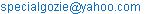Acha Chigozie Kelechi. Rescaling Residual Bootstrap and Wild Bootstrap. International Journal of Data Science and Analysis. Vol. 2, No. 1, 2016, pp. 7-14. doi: 10.11648/j.ijdsa.20160201.12

Received: July 20, 2016; Accepted: October 14, 2016; Published: October 28, 2016

Abstract: This paper examines and discusses a comparative analysis of hypothetical data by using bootstrap methods. The residual and wild bootstrap methods, including their rescaled versions were applied on the data collected from a normal distribution with different ability levels to check whether they are significant at various assessment conditions. The wild bootstrap compared in this paper are from Mammen and Redamarche distributions. In addition their kernel density plot is used to ascertain the trends and the performance at the lower ends of the distributions for each bootstrap model and also the trend as sample size tends to infinity. To achieve this, each of the forms were represented by using at least one functional model each from hypothetical data sets of a particular bootstrap data generating process (DGP) method to illustrate how 8640 scenerios were estimated. The result shows that the Hypothetical Rescaled Residual (HRR) is found to be preferable to the Hypothetical Unrescaled Residual (HR) while Hypothetical Wild Redamarche Model (HRWR) is found to be preferable to the Hypothetical Wild Mammen model (HRWM) with reference to their bias, standard error and root mean square error (RMSE) at different levels of significance, that is, B=99, N(0,1), n1 & n3 = 10000, RMSE = -0.0004 &-0.0025 respectively. Aslo, B=99, N(0,1), n3 = 10000, RMSE = -0.0004. Even though at B=99, N(0,1), n2 = 10000, RMSE for HRWM (0.0601) is higher than HRWR (0.0595). In fact, across all the models, rescaled residual functional model out performed all other functional models considered in this paper. Also, the trends at the lower ends of the distributions for each bootstrap model shows that the empirical distributions of true distributions follow the chi-square distribution and also tends to normal distribution as sample size tends to inifinity.

Keywords: Rescaled, Bootstrap, Hypothetical Models, Mammen Distributions, Redamarche Distributions

Contents

1. Introduction

The basic idea of bootstrap testing is that, when a test statistic of interest has an unknown distribution, that distribution can be characterized by using information in the data set that is being analyzed. Bootstrapping is the practice of estimating properties of an estimator by measuring those properties when sampling from an approximating distribution. One standard choice for an approximating distribution is the empirical distribution of the observed data. This can give to models which can be restricted and unrestricted. When something is unrestricted, it means there are no restrictions placed on it. A restriction is a rule about a way that something can be used. It is a new definition that has been introduced, to define the sensitivity of a particular variable. It is also, a limitation which cannot be exceeded or rules which cannot be broken.

In this research, restricted models will be considered and for a model to restricted; it will be rescaled. Rescaling is mathematical operation that changes the measurement scale of a variable, stabilizing variance, normalizing, and linearizing a relationship.

Given,

yt =X tβ + µ t; E(µt/Xt) = 0, E(µSµ t = 0)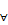s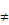t, µt ~NID (0; σ2) (1)

where the dependent variable, yt is a linear combination of the parameters (but need not be linear in the independent variables), n is the number of observations, β is a k-vector, and the 1 × k vector of regressors X t, which is the tth row of the n × k matrix X, is treated as fixed and µ is an n × 1 vector of independent identically distributed errors with mean 0 and variance σ2. The true distribution of µ is not known.

The corresponding dependent variables from the bootstrap methods are given by;

yb = Xβ* + et                                   (2)

For each vector yb the estimator is recomputed and the sampling distribution of the estimator is estimated by the assumed distribution and empirical distribution respectively, of these estimates computed over a large number of yb.

Generally, restriction on the models makes bootstrap tests more reliable, because the parameters of the bootstrap DGP are estimated more precisely. The interest in this study was ignited by , ; , which called for more research on the parametric bootstrap method and for comparative studies of parametric and nonparametric approaches. Therefore, This paper examines and discusses a comparative analysis on hypothetical data by using bootstrap methods. In this study, the residual and wild bootstrap methods, including their rescaled versions were applied on the data collected from a normal distribution with different ability levels to check whether they are significant at various assessment conditions. In addition their kernel density plot is used to ascertain the trends and the performance at the lower ends of the distributions for each bootstrap model. To achieve this, each of the forms were represented by using at least one functional model each from hypothetical data sets of a particular bootstrap data generating processes (DGPs) method to illustrate how 8640 scenerios were estimated. In addition to the standard errors (precision), bias and root mean square error, the trends of the bootstrap distribution methods of the sample will be established from a normal distribution of different forms as sample size tends to infinity.

2. Literature Review

, established that there are many bootstrap methods that can be used for econometric analysis, such as, regression models with independent and identical distribution (iid). , , in their paper "The wild bootstrap, tamed at last", discovered that based on an extensive Monte Carlo study on the spatial and cross- sectional data, the wild bootstrap test was proposed for the evaluation of linear regression models based on restricted residuals. Also, the wild bootstrap consistently performs better than non-bootstrap heteroskedasticity consistent covariance matrix (HCCM) - based methods. Critical values for the tests than asymptotic theory was used by several authors including , , , , , , , , , , ), , in the linear regression estimation. , studied the efficiency of the Residual and Parametric Bootstrap Techniques. , after augmenting the data with normal underlying variables, compute with Gaussian distribution for latent variable using parametric bootstrap method. Also, the bit regression algorithm of , was included in the computation. This function generates a sample from the posterior distribution of a probit regression model using the data augmentation approach of .  key insight was to observe that after pivoting  once, it can improve the conﬁdence intervals for the mean. Other authors like  applied non-parametric data dependent bootstrap for conditional moment model in simple linear regression.  in his study of boostrap used simulation methods for bayesian econometric models: inference, development and communication. For example, many authors applied Geweke's spectral bootstrap measures to describe causal interactions among different areas in the linear regression of an unknown parameter. Using simulation methods for regression, each group of parameters and latent variables is simulated conditional on all the others ; . Bootstrap and Other Resampling Methods in Regression Analysis was discussed in detail by .  studied the exact likelihood analysis of the multinomial probit and normal regression models. To evaluate the inference in the regression models of representative samples, a number of authors have studied the single period case, , and ). ;  emphasis on the importance of acurrate statistical inference on different bootstrap data generating functional models.  gives a thorough review and comparison of the approaches in calculating standard errors in many areas of study including econometrics, regression, statistics, biometrics and so on.

3. Research Methodology

The hypothetical data set for this paper was collected from Normal distribution of disferrent ability levels under various assessment conditions using bootstrap methods;

A. The residual bootstrap method whose algorithm is as follows;

i.      Fit the model, retain fitted values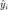and the residuals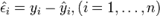.

ii.     Create synthetic response variables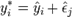where j is selected randomly from the list (1, …, n) for every i.

iii.   Refit the model using the fictitious response variables y*i, and retain the quantities of the parameters,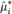, estimated from the synthetic y*i.

iv.   Repeat steps 2 and 3 a statistically significant number of times.

Unless the quantity to be bootstrapped is invariant to the variance of the error terms, if not, it is advisable to rescale the residuals so that they have the correct variance. The simplest type of rescaled residual is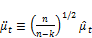(3)

The first factor here is the inverse of the square root of the factor by which 1/n times the sum of squared residuals underestimates σ2. A somewhat more complicated method uses the diagonals of the ‘hat matrix’

X(XTX)−1XT    (4)

to rescale each residual by a different factor, which will be adopted in this paper. The residual bootstrap DGP using rescaled residuals generates a typical observation of the bootstrap sample by the equation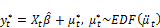(5)

The bootstrap errors here are said to be ‘resampled’ from the empirical distribution function, or EDF, of the üt. This function assigns probability 1/n to each of the üt. Thus, each of the bootstrap error terms can take on n possible values, namely, the values of the üt, each with probability 1/n.

B. The wild bootstrap

The residual bootstrap is not valid if the error terms are not independently and identically distributed, but two other commonly used bootstrap methods are valid in this case. The first of these is the ‘wild bootstrap’, which was proposed by  for regression models. The idea of wild bootstrap is, like the residual bootstrap, to leave the regressors at their sample value, but to resample the response variable based on the residuals values. That is, for each replicate, one computes a new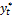. For a model like (5) with independent but possibly heteroskedastic errors, the wild bootstrap DGP is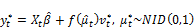(6)

where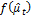is a rescaled form of the tth residual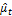, and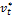is a random variable with mean 0 and variance 1. One possible choice for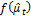is just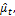but a better choice is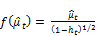(7)

where ht is the tth diagonal of the ‘hat matrix’ (4). According to , When theare defined by (7), they would have constant variance if the error terms were homoskedastic. There are various ways to specify the distribution of the. This method assumes that the 'true' residual distribution is symmetric and can offer advantages over simple residual sampling for smaller sample sizes. Different forms are used for the random variable, but two forms will be considered;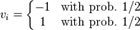(8)

The Mammen distribution, ; :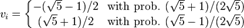(9)

NOTATIONS

HR - Hypothetical Residual Functional Model;

HRR - Hypothetical Rescaled Residual Functional Model;

HRWR - Hypothetical Rescaled Wild Functional Model from the Rademacher distribution;

HRWM - Hypothetical Rescaled Wild Functional Model from the Mammen distribution.

4. Analysis and Discussion of Results

Under this section the bootstrap methods applied are the residual bootstrap and wild bootstrap. Each of the forms were represented by using at least one functional model each from hypothetical data sets of a particular bootstrap DGP method to illustrate how the values in tables 1, 2, and 3.

Recall (1), yb = Xβ* + et: which is equivalent to (10) will be used to estimate original hypothetical data sets with fixed sample size.

i.      The results gotten from the Unrescaled Residual bootstrap when applied on the hypothetical data sets with fixed sample size is as follows;:

Hypothetical Model: SLR Equation Estimated from the Unrescaled Residual bootstrap:

HYPt = bo + b1A+ b2B+ et                     (10)

Hypothetical Unrescaled Residual Model (HR), B=499, N(,0.9), n2=1000:

HYPt = 24.42316 b1 + 0.06562462 b2          (11)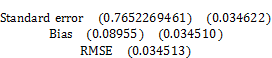ii.     The results gotten from the rescaled residual bootstrap when applied on the hypothetical data sets with fixed sample size is as follows;

The residual bootstrap DGP using rescaled residuals(12)

where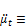X(XTX)−1XT

Hypothetical data set: SLR Equation Estimated from (12) Rescaled Residual Model (HRR), B=499, N(0,0.9), n2=1000;

HYPt = 24.14231687 b1 + 0.05696246 b2       (13)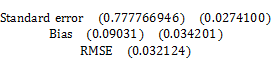iii.   The results gotten from the wild bootstrap DGP when applied on the hypothetical data sets with fixed sample size is as follows;

The wild bootstrap DGP is(14)

where(15)

Rescaled wild model (HRWR) from Rademacher distribution B=499, N(0,0.9), n2=1000,

using (14)

HYPt = 23.94231687 b1 + 0.04696246 b2        (16)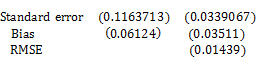Rescaled wild model (HRWM) from Mammen distribution B=499, N(0,0.9), n2=10000 using (14)

HYPt = 23.8652b1 + 0.04145213 b2              (17)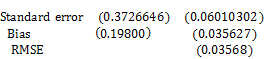Majority of the interest on this paper lies on the RMSE (Table A3) since it is the square root of the squared bias and squared standard error put together.

5. Summary and Interpretation of Results

The interpretation of the Bias, Standard Error and Root Mean Square Error estimated from a hypothetical data set will enable determine the present the effects of the factors of sample size and bootstrap level. Extreme values in the ranges stated above were truncated and special consideration was given to the plotting range and the layout. Even though very low estimates were also observed, results in these ranges are presented in order to demonstrate the trends and the performance at the lower ends of the distributions for each bootstrap model.

Table (A1-A3) shows the bias, standard error and root mean square respectively, of the three ability levels for the bootstrap. In fact, two cases were considered first-correlation between residual original values and rescaled residual values; secondly- correlation between wild values and wild values from different distributions were considered. Although the magnitude of bias varied across the bootstrap methods (HR, HRR, HRWR, and HRWM), the pattern of relative effects of these factors was generally consistent within each bootstrap method (or model). It can be seen that sample size and test length of bootstrap level had large effects on RMSE (which takes care of the bias and standard error) of the simple linear regression (SLR). The ability levels had relatively small or mixed effects under various assessment conditions. RMSE was smaller for larger sample sizes and bootstrap levels, especially in HRR.

It can also be seen that, across different combinations, that as different test lengths (n1, n2, n3) of ability levels, and the sample size increased, the bias obtained from all the bootstrap models; decreased at almost all estimated points, which is to be expected because of the property of estimation bias. It can also be noted that although the RMSE at the two ends of the estimate was large (in absolute value), the curves from different bootstrap models were closer to one another when the sample size was 10000 than when the sample size was 10. Across all the conditions considered, models HRWM and HRWR yielded much larger RMSE than the other models at almost all the estimates. Model HRWM produced the largest RMSE. The smallest and the second smallest bias were associated with models HRR and HR across almost all estimates. Therefore, for the bootstrap models, the pattern was clear that lower sample sizes and bootsrap levels were associated with larger RMSE while higher sample sizes, rescaled were related to lower bias. This is not surprising, because the fitted distribution with the higher sample sizes even when bootstrapped was more similar to the distribution of the original data. However, it does not mean the higher sample sizes were always associated with the smaller bias along all the estimated values. It is pertanent to note that the difference between the models are so large, not to be approximately used for further prediction (see Table 3). For example, B=99, N(0,1), n1 & n3 = 10000, RMSE = -0.0004 &-0.0025 respectively. Aslo, B=99, N(0,1), n3 = 10000, RMSE = -0.0004. Even though at B=99, N(0,1), n2 = 10000, RMSE for HRWM(0.0601) is higher than HRWR (0.0595). HRWR still have the minimum across all other points of estimation under various assessment conditions. The possible reason could be that model HRWR was over smoothing in that parameter estimates. As to the factor of ability levels, no substantial effect on bias was observed across the conditions examined. A general observation is that across different group proficiency levels, as the sample size, bootstrap level increased, the bias reduce meanwhile, the differences among the different restricted parametric bootstrap models were becoming more similar. The corresponding figures (B1-B3) provide information to evaluate the relative effects of sample size, bootstrap level and ability levels on the bias, standard error and RMSE of the SLR, which reveals the distribution of hypothetical data set to be a Chi- Square distribution.

Interpretation of the hypothetical data set in econometric terms; using the restricted models, for example the (4.4) - HRR result indicates positive relationship between HYPt and b2. The positive sign of the b1 shows a great improvement in the HYPt dataset as suggested by economic theory. The high coefficient of determination and multiple R-squared shows that the model is a reasonable fit of the relationship among the variables. It also confirms its efficiency in prediction. The alternative hypothesis that the hypothetical data set is significant is accepted. The best set of parameters found in MLE are under the function that is minimized with first argument and the vector of parameters over which minimization is to take place, which makes the result a scalar. Since, convergence is one, this indicates that the iteration limit matrix had been reached. Likewise, the other hypothetical rescaled models follow the same pattern.

6. Conclusion

The result shows that the rescaled residual method using the diagonals of the hat matrix is a bit better than all other functional bootstrap models considered in this paper when some observations have high leverage. Though, leverage in regression analyses aimed at identifying those observations that are far away from corresponding average predictor values. It is good to note that leverage points do not necessarily have a large effect on the outcome of fitting regression models. The result have also shown that wild bootstrap tests based on Rademacher distribution usually perform better than wild bootstrap tests that use Mammen distribution which is in conformity with the result gotten by  especially when the conditional distribution of the error terms is approximately symmetric. Both methods seems to perform best when n is very large. Generally, inference based on rescaled residual (RR) is extra-ordinary reliable but RR performed quite poorly when sample size (n) is small. Even though very low estimates were also observed, results in these ranges are presented in order to demonstrate the trends and the performance at the lower ends of the distributions for each bootstrap model using the kernel density plot. The results of the plots at the lower ends of the distributions for each bootstrap model showed that hypothetical data set is a Chi-square distribution. Figure B3 represents the behavior of the hypothetical data set as sample size tend to infinity under various assessment conditions and it shows that the data set tends to a normal distribution and a very suitable platform for precision and further research works. This paper suggest that further studies should carried out to verify whether there was an interaction effect between the factor and the bootstrap DGP method considered in this paper.

Appendices

Appendix A: Tables A1-A3

Table A1. Comparison of Bias in the SLR for Parametric Bootstrap Models in a hypothetical data set.

 bootstrap Level Ability Level Sample Size Bootstrap Models Bootstrap Models HR HRR Diff HRWM HRWR Diff B=99 N(0,1) n1 200 0.1224 0.1224 0.0000 0.1189 0.1190 - 0.0001 1000 0.0319 0.0318 0.0001 0.0498 0.0495 0.0003 10000 0.0160 0.0164 -0.0006 0.0337 0.0337 0.0000 n2 200 0.0783 0.0781 -0.0002 0.0812 0.0816 -0.0004 1000 0.0204 0.0203 0.0001 0.0647 0.0647 0.0000 10000 0.0344 0.0342 -0.0002 0.0165 0.0168 0.0003 n3 200 0.0829 0.0824 0.0005 0.1240 0.1236 0.0004 1000 0.0356 0.0353 0.0003 0.0601 0.0603 -0.0002 10000 0.0177 0.0176 0.0001 0.0331 0.0332 -0.0001 B=499 N(0,0.9) n1 200 0.0224 0.0226 -0.0002 0.1197 0.1196 0.0001 1000 0.0259 0.0258 0.0001 0.0336 0.0336 0.0000 10000 0.0329 0.0326 0.0003 0.0165 0.0164 0.0001 n2 200 0.0765 0.0763 -0.0002 0.0812 0.0809 0.0003 1000 0.0597 0.0597 0.0000 0.0347 0.0351 -0.0004 10000 0.0334 0.0330 0.0004 0.0166 0.0166 0.0000 n3 200 0.0748 0.0747 -0.0001 0.0829 0.0829 0.0000 1000 0.0345 0.0342 -0.0003 0.0356 0.0351 0.0005 10000 0.0331 0.0330 0.0001 0.0177 0.0175 0.0002 B=1999 N(1,0.25) n1 200 0.0813 0.0812 0.0001 0.0885 0.0880 0.005 1000 0.0333 0.0333 0.0000 0.0352 0.0349 0.003 10000 0.0172 0.0170 0.0002 0.0173 0.0173 0.000 n2 200 0.0828 0.0826 0.0002 0.1048 0.1048 0.000 1000 0.0332 0.0332 -0.0000 0.0355 0.0356 -0.0001 10000 0.0170 0.0171 0.0001 0.0267 0.0267 0.0000 n3 200 0.0814 0.0814 0.0000 0.1032 0.1030 0.0002 1000 0.0308 0.0306 0.0002 0.0565 0.0568 -0.0003 10000 0.0289 0.0286 -0.0003 0.0176 0.0176 0.0000

Note. Bold values used as examples in the paper.

Table A2. Comparison of Standard Error of the SLR for Parametric Bootstrap Models in a hypothetical data set.

 bootstrap Level Ability Level Sample Size Bootstrap Models Bootstrap Models HRR311A R311H Diff HWRR HWRM Diff B=99 N(0,1) n1 200 0.0813 0.0744 0.0069 0.0885 0.0891 -0.0006 1000 0.0333 0.0279 0.0054 0.0352 0.0352 0.0000 10000 0.0172 0.0129 0.0043 0.0173 0.0173 0.0000 n2 200 0.0828 0.0758 0.0070 0.1048 0.1049 -0.0001 1000 0.0332 0.0270 0.0062 0.0355 0.0359 -0.0004 10000 0.0170 0.0130 0.0040 0.0267 0.0268 -0.0001 n3 200 0.0814 0.0729 0.0085 0.1032 0.1042 -0.0010 1000 0.0308 0.0236 0.0072 0.0565 0.0563 0.0002 10000 0.0289 0.0252 0.0037 0.0176 0.0178 -0.0002 B=499 N(0,0.9) n1 200 0.1224 0.1036 0.0188 0.1189 0.1139 0.0050 1000 0.0311 0.0248 0.0063 0.0498 0.0443 0.0055 10000 0.0140 0.0038 0.0102 0.0337 0.0307 0.0030 n2 200 0.0783 0.0737 0.0046 0.0312 0.0121 0.0191 1000 0.0204 0.0150 0.0054 0.0647 0.0573 0.0074 10000 0.0340 0.0312 0.0028 0.0165 0.0067 0.0098 n3 200 0.0829 0.0638 0.0191 0.1240 0.1193 0.0047 1000 0.0356 0.0274 0.0082 0.0601 0.0539 0.0062 10000 0.0177 0.0075 0.0102 0.0331 0.0301 0.0030 B=1999 N(1,0.25) n1 200 0.1224 0.1165 0.0059 0.1197 0.0985 0.0212 1000 0.0598 0.0537 0.0061 0.0336 0.0248 0.0088 10000 0.0329 0.0296 0.0033 0.0165 0.0052 0.0113 n2 200 0.0765 0.0549 0.0216 0.0812 0.0760 0.0052 1000 0.0597 0.0498 0.0099 0.0347 0.0287 0.0060 10000 0.0334 0.0225 0.0109 0.0166 0.0135 0.0031 n3 200 0.0748 0.0691 0.0057 0.0829 0.0612 0.0217 1000 0.0345 0.0277 0.0068 0.0356 0.0248 0.0108 10000 0.0331 0.0298 0.0033 0.0177 0.0163 0.0114

Note. Bold values used as examples in the paper.

Table A3. Comparison of RMSE of the SLR for Parametric Bootstrap Models in a hypothetical data set.

 bootstrap Level Ability Level Sample Size Bootstrap Models Bootstrap Models HRR311A R311H Diff HWRM HWRR Diff B=99 N(0,1) n1 200 0.1224 0.1228 -0.0004 0.1189 0.1114 0.0075 1000 0.0119 0.0025 0.0094 0.0498 0.0489 0.0009 10000 0.0120 0.0099 0.0021 0.0337 0.0294 0.0043 n2 200 0.0783 0.0706 0.0077 0.0812 0.0768 0.0044 1000 0.0404 0.0391 0.0013 0.0647 0.0530 0.0117 10000 0.0340 0.0294 0.0056 0.0165 0.0169 -0.0004 n3 200 0.0829 0.0854 -0.0025 0.1240 0.1154 0.0086 1000 0.0356 0.0233 0.0123 0.0601 0.0595 0.0006 10000 0.0177 0.0164 0.0013 0.0331 0.0284 0.0047 B=499 N(0,0.9) n1 200 0.1224 0.1106 0.0118 0.1197 0.1101 0.0096 1000 0.0598 0.0575 0.0023 0.0336 0.0133 0.0203 10000 0.0399 0.0339 0.0060 0.0165 0.0113 0.0052 n2 200 0.0765 0.0708 0.0057 0.0812 0.0699 0.0113 1000 0.0597 0.0389 0.0208 0.0347 0.0321 0.0026 10000 0.0334 0.0297 0.0037 0.0166 0.0097 0.0069 n3 200 0.0748 0.0611 0.0137 0.0829 0.0746 0.0083 1000 0.0345 0.0321 0.0024 0.0356 0.0143 0.0213 10000 0.0331 0.0263 0.0068 0.0177 0.0130 0.0047 B=1999 N(1,0.25) n1 200 0.1063 0.0993 0.0070 0.0885 0.0819 0.0066 1000 0.0518 0.0460 0.0058 0.0352 0.0331 0.0021 10000 0.0297 0.0249 0.0048 0.1048 0.1029 0.0019 n2 200 0.0828 0.0774 0.0054 0.0173 0.0135 0.0038 1000 0.0332 0.0309 0.0023 0.0355 0.0323 0.0030 10000 0.0170 0.0126 0.0044 0.0267 0.0235 0.0032 n3 200 0.0714 0.0663 0.0051 0.1032 0.0968 0.0064 1000 0.0318 0.0263 0.0055 0.0565 0.0544 0.0021 10000 0.0018 -0.0022 0.0040 0.0176 0.0132 0.0044

Note. Bold values used as examples in the paper.

Appendix B: Tables B1-B3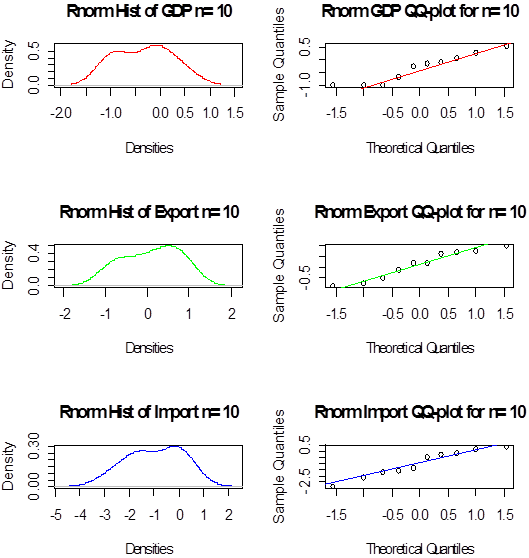Figure B1. Kernel Density Plot representing the behavior of the Data Set as Sample Size Equals to Ten Under Various Assessment Conditions.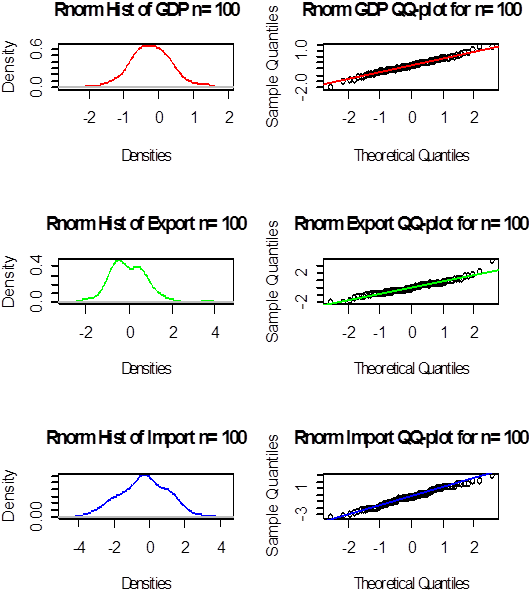Figure B2. Kernel Density Plot Representing the Behavior of the Data Set as Sample Size equals to one Hundred Under Various Assessment Conditions.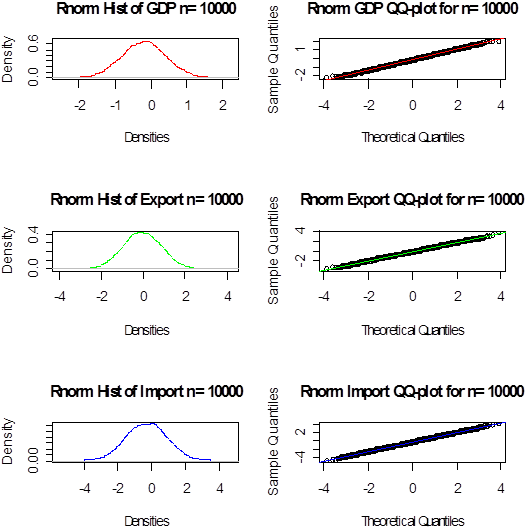Figure B3. Kernel Density Plot representing the behavior of the Data Set as Sample Size tend to Infinity Under Various Assessment Conditions.

References

1. Acha, C. K. (2014) Parametric Bootstrap Methods for Parameter Estimation in SLR Models. International Journal of Econometrics and Financial Management, 2(5), 175–179. Doi:10.12691/ijefm-2-5-2.
2. Acha, C. K. (2014) Bootstrapping Normal and Binomial Distributions. International Journal of Econometrics and Financial Management, 2(6), 253– 256. Doi:10.12691/ijefm-2-6-2.
3. Acha, C.K. and Acha I.A. (2015) Smooth Bootstrap Methods on External Sector Statistics. International Journal of Econometrics and Financial Management, 3(3), 115–120. Doi:10.12691/ijefm-3-3-2.
4. MacKinnon, J.G., (2006). Bootstrap Methods in Econometrics, The Economic Record, The Economic Society of Australia, 82(1), 2-18.
5. Davidson, R. and Flachaire, E. (2001), The Wild Bootstrap,Tamed at Last, GREQAM Document de Travail 99A32, revised.
6. Davidson, R. and Flachaire, E.,(2008). The wild bootstrap, tamed at last, Journal of Econometrics, Elsevier, 146(1), 162-169.
7. Lahiri, S. N. (2006). Bootstrap Methods: A Review. In Frontiers in Statistics (J. Fan and H.L. Koul, editors) 231-265, Imperial College Press, London.
8. Flachaire, E. (2005). More efficient tests robust to heteroskedasticity of unknown form.Econ. Rev.24,219–241.
9. Godfrey, L. G. (1998). Tests of non-nested regression models: Some results on small sample behaviour and the bootstrap, Journal of Econometrics, 84, 59–74.
10. Godfrey,L. G., andVeall, M. R. (2000). Alternative approaches to testing by variable addition.Econ. Rev.19, 241–261.
11. Davidson, R. and MacKinnon, J.G. (1996). The Power of Bootstrap Tests, Working Papers 937, Queen's University, Department of Economics.
12. Davidson, R & MacKinnon, J. G. (1999). The Size Distortion of Bootstrap Tests, Econometric Theory, Cambridge University Press, vol. 15(03), pages 361-376, June.
13. Davidson, R. and MacKinnon, J.(2000). Bootstrap tests: how many bootstraps?, Econometric Reviews, Taylor and Francis Journals, 19(1), 55-68.
14. Davidson, R. and MacKinnon, J.G., (2006a). The power of bootstrap and asymptotic tests, Journal of Econometrics, Elsevier, 133(2), 421-441.
15. Davidson, R. and MacKinnon, J.G. (2006b), ‘Bootstrap Methods in Econometrics’, in Patterson, K. and Mills, T.C. (eds), Palgrave Handbook of Econometrics: Volume 1 Theoretical Econometrics. Palgrave Macmillan, Basingstoke; 812–38.
16. Acha, I. A. and Acha, C. K. (2011). Interest Rates in Nigeria: An Analytical Perspective. Research Journal of Finance and Accounting, 2(3); 71-81 www.iiste.org ISSN 2222-1697 (Paper) ISSN 2222-2847 (Online).
17. Acha, C. K. and Omekara, C. O. (2016) Towards Efficiency in the Residual and Parametric Bootstrap Techniques. American Journal of Theoretical and Applied Statistics. 5(5) 285-289. doi: 10.11648/j.ajtas.20160505.16.
18. Albert, J., and Chib, S. (1993) ‘Bayes inference via Gibbs sampling of autoregressive time series subject to Markov mean and variance shifts", Journal of Business and Economic Statistics 11, 1–15.
19. Beran, R. (1988), ‘Prepivoting Test Statistics: A Bootstrap View of Asymptotic Refinements’, Journal of the American Statistical Association, 83, 687–97.
20. Hansen, B. E. (2000). Testing for structural change in conditional models.J. Econ.97, 93–115.
21. Geweke, J. (1999) ‘Using simulation methods for Bayesian econometric models: Inference, development and communication’ (with discussion and reply), Econometric Reviews 18, 1–126.
22. Wu, C. F. J. (1986). Jackknife, bootstrap and other resampling methods in regression analysis. Annals of. Statistics. 14, 1261-1295.
23. McCulloch, R. and P. Rossi, P. (1994) ‘An exact likelihood analysis of the multinomial probit model,’ Journal of Econometrics 64, 207–40.
24. MacKinnon, J. G. and Smith, A.A. (1998). Approximate bias correction in econometrics, Journal of Econometrics, Elsevier, 85(2), 205-230.
25. MacKinnon J. G. (2002), Bootstrap inference in econometrics, Canadian Journal of Economics Revue Canadienne dEconomique, 35(4): 615-645. On-line: 10.1111/0008-4085.00147.
26. Mammen, E. (1992). Bootstrap and wild bootstrap for high dimensional linear models.Ann. Statist.21, 255–285.
27. Mammen, E. (1993). Bootstrap and wild bootstrap for high dimensional linear models.Ann. Statist.21, 255–285.

 Contents 1. 2. 3. 4. 5. 6.
Article ToolsAbstractPDF(904K)